TypeScript 4.0正式发布！现在是开始使用它的最佳时机https://www.typescriptlang.org/

TypeScript 4.0 没有引入特别重大的更改。实际上，如果你刚刚开始接触这种语言，那么现在是开始使用它的最佳时机。它的社区已经成熟完善，并在不断发展，拥有可运行的代码和很棒的新资源可供学习。还有一件事情：尽管我们为 4.0 引入了那么多好东西，但你实际上只需要了解 TypeScript 的基础知识就可以开始生产应用了！

``````npm install -D typescript
``````

4.0 之旅

TypeScript 是当今许多人的 JavaScript 技术栈的核心部分。在 npm 上，TypeScript 在 7 月首次实现了超过 5000 万的月下载量！尽管我们知道它总有增长和改进的余地，但很明显，大多数使用 TypeScript 编码的开发人员确实很喜欢它。StackOverflow 的最新开发人员调查将 TypeScript 列为第二受欢迎的语言。在最新的 JS 现状调查中，使用 TypeScript 的开发人员中有大约 89% 表示会再次使用它。

TypeScript 3.1 扩展了映射类型的功能以处理元组和数组类型，并极大简化了将属性附加到函数的过程，而无需使用 TypeScript 专属的运行时功能（已停用）。

TypeScript 3.2 允许对象在泛型类型上传播，并通过严格类型化 bind、call 和 apply，利用 3.0 的功能更好地建模函数的元编程。TypeScript 3.3 更多关注稳定性，但也改进了联合类型方法，并在 --build 模式下添加了文件增量式构建。

TypeScript 3.7 是一个非常值得关注的版本，因为它很好地结合了许多新的类型系统特性与 ECMAScript 特性。在类型系统方面，我们加入了递归类型别名引用和对断言样式函数的支持，这两者都是独特的类型系统特性。从 JavaScript 方面来看，该版本带来了可选链和空值合并功能，这是 TypeScript 和 JavaScript 用户最期待的两项功能。

``````function concat(arr1, arr2) {    return [...arr1, ...arr2];}
``````

``````function tail(arg) {    const [_, ...result] = arg;    return result}
``````

``````function concat<>(arr1: [], arr2: []): [A];function concat<A>(arr1: [A], arr2: []): [A];function concat<A, B>(arr1: [A, B], arr2: []): [A, B];function concat<A, B, C>(arr1: [A, B, C], arr2: []): [A, B, C];function concat<A, B, C, D>(arr1: [A, B, C, D], arr2: []): [A, B, C, D];function concat<A, B, C, D, E>(arr1: [A, B, C, D, E], arr2: []): [A, B, C, D, E];function concat<A, B, C, D, E, F>(arr1: [A, B, C, D, E, F], arr2: []): [A, B, C, D, E, F];)
``````

``````function concat<A2>(arr1: [], arr2: [A2]): [A2];function concat<A1, A2>(arr1: [A1], arr2: [A2]): [A1, A2];function concat<A1, B1, A2>(arr1: [A1, B1], arr2: [A2]): [A1, B1, A2];function concat<A1, B1, C1, A2>(arr1: [A1, B1, C1], arr2: [A2]): [A1, B1, C1, A2];function concat<A1, B1, C1, D1, A2>(arr1: [A1, B1, C1, D1], arr2: [A2]): [A1, B1, C1, D1, A2];function concat<A1, B1, C1, D1, E1, A2>(arr1: [A1, B1, C1, D1, E1], arr2: [A2]): [A1, B1, C1, D1, E1, A2];function concat<A1, B1, C1, D1, E1, F1, A2>(arr1: [A1, B1, C1, D1, E1, F1], arr2: [A2]): [A1, B1, C1, D1, E1, F1, A2];
``````

``````function concat<T, U>(arr1: T[], arr2, U[]): Array<T | U>;
``````

``````function tail<T extends any[]>(arr: readonly [any, ...T]) {    const [_ignored, ...rest] = arr;    return rest;}const myTuple = [1, 2, 3, 4] as const;const myArray = ["hello", "world"];// type [2, 3, 4]const r1 = tail(myTuple);// type [2, 3, ...string[]]const r2 = tail([...myTuple, ...myArray] as const);
``````

``````type Strings = [string, string];type Numbers = [number, number];// [string, string, number, number]type StrStrNumNum = [...Strings, ...Numbers];
``````

``````A rest element must be last in a tuple type.
``````

``````type Strings = [string, string];type Numbers = number[]// [string, string, ...Array<number | boolean>]type Unbounded = [...Strings, ...Numbers, boolean];
``````

``````type Arr = readonly any[];function concat<T extends Arr, U extends Arr>(arr1: T, arr2: U): [...T, ...U] {    return [...arr1, ...arr2];}
``````

``````function partialCall(f, ...headArgs) {    return (...tailArgs) => f(...headArgs, ...tailArgs)}
``````

TypeScript 4.0 改进了 rest 参数和 rest 元组元素的推断过程，因此我们可以类型化它并使其“正常工作”。

``````type Arr = readonly unknown[];function partialCall<T extends Arr, U extends Arr, R>(f: (...args: [...T, ...U]) => R, ...headArgs: T) {    return (...b: U) => f(...headArgs, ...b)}
``````

``````const foo = (x: string, y: number, z: boolean) => {}// This doesn't work because we're feeding in the wrong type for 'x'.const f1 = partialCall(foo, 100);// ~~~// error! Argument of type 'number' is not assignable to parameter of type 'string'.// This doesn't work because we're passing in too many arguments.const f2 = partialCall(foo, "hello", 100, true, "oops")// ~~~~~~// error! Expected 4 arguments, but got 5.// This works! It has the type '(y: number, z: boolean) => void'const f3 = partialCall(foo, "hello");// What can we do with f3 now?f3(123, true); // works!f3();// error! Expected 2 arguments, but got 0.f3(123, "hello");// ~~~~~~~// error! Argument of type '"hello"' is not assignable to parameter of type 'boolean'.
``````

``````function foo(...args: [string, number]): void {    // ...}
``````

``````function foo(arg0: string, arg1: number): void {    // ...}
``````

``````foo("hello", 42); // worksfoo("hello", 42, true); // errorfoo("hello"); // error
``````

``````type Range = [start: number, end: number];
``````

``````type Foo = [first: number, second?: string, ...rest: any[]];
``````

``````type Bar = [first: string, number];// ~~~~~~// error! Tuple members must all have names or all not have names.
``````

``````function foo(x: [first: string, second: number]) {    // ...    // note: we didn't need to name these 'first' and 'second'    let [a, b] = x;    // ...}
``````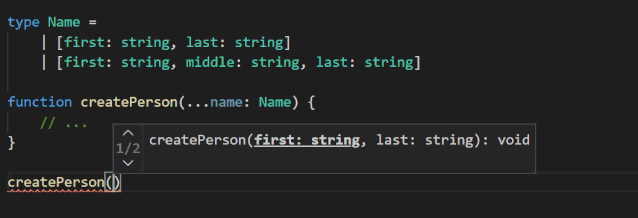https://github.com/microsoft/TypeScript/pull/38234

``````class Square {    // Previously: implicit any!    // Now: inferred to `number`!    area;    sideLength;    constructor(sideLength: number) {        this.sideLength = sideLength;        this.area = sideLength ** 2;    }}
``````

``````class Square {    sideLength;    constructor(sideLength: number) {        if (Math.random()) {            this.sideLength = sideLength;        }    }    get area() {        return this.sideLength ** 2;        // ~~~~~~~~~~~~~~~        // error! Object is possibly 'undefined'.    }}
``````

``````class Square {    // definite assignment assertion    // v    sideLength!: number;    // ^^^^^^^^    // type annotation    constructor(sideLength: number) {        this.initialize(sideLength)    }    initialize(sideLength: number) {        this.sideLength = sideLength;    }    get area() {        return this.sideLength ** 2;    }}
``````

JavaScript 和许多语言都支持一组称为"复合赋值运算符"的运算符。复合赋值运算符将一个运算符应用于两个参数，然后将结果赋给左侧。你可能以前看过这些：

``````// Addition// a = a + ba += b;// Subtraction// a = a - ba -= b;// Multiplication// a = a * ba *= b;// Division// a = a / ba /= b;// Exponentiation// a = a ** ba **= b;// Left Bit Shift// a = a << ba <<= b;
``````

JavaScript 中有很多运算符都有对应的赋值运算符！但是有三个值得注意的例外：逻辑和（&&），逻辑或（||）和空值合并（??）。

``````a = a && b;a = a || b;a = a ?? b;
``````

``````// could be 'a ||= b'if (!a) {    a = b;}
``````

``````let values: string[];// Before(values ?? (values = [])).push("hello");// After(values ??= []).push("hello");
``````

``````obj.prop ||= foo();// roughly equivalent to either of the followingobj.prop || (obj.prop = foo());if (!obj.prop) {    obj.prop = foo();}
``````

``````const obj = {    get prop() {        console.log("getter has run");        // Replace me!        return Math.random() < 0.5;    },    set prop(_val: boolean) {        console.log("setter has run");    }};function foo() {    console.log("right side evaluated");    return true;}console.log("This one always runs the setter");obj.prop = obj.prop || foo();console.log("This one *sometimes* runs the setter");obj.prop ||= foo();
``````

https://github.com/microsoft/TypeScript/pull/37727

catch 子句绑定支持 unknown

``````try {    // ...}catch (x) {    // x has type 'any' - have fun!    console.log(x.message);    console.log(x.toUpperCase());    x++;    x.yadda.yadda.yadda();}
``````

``````try {    // ...}catch (e: unknown) {    // error!    // Property 'toUpperCase' does not exist on type 'unknown'.    console.log(e.toUpperCase());    if (typeof e === "string") {        // works!        // We've narrowed 'e' down to the type 'string'.        console.log(e.toUpperCase());    }}
``````

https://github.com/microsoft/TypeScript/pull/39015

``````{  "compilerOptions": {    "target": "esnext",    "module": "commonjs",    "jsx": "react",    "jsxFactory": "h",    "jsxFragmentFactory": "Fragment"  }}
``````

``````// Note: these pragma comments need to be written// with a JSDoc-style multiline syntax to take effect./** @jsx h *//** @jsxFrag Fragment */import { h, Fragment } from "preact";let stuff = <>    <div>Hello</div></>;
``````

``````// Note: these pragma comments need to be written// with a JSDoc-style multiline syntax to take effect./** @jsx h *//** @jsxFrag Fragment */import { h, Fragment } from "preact";let stuff = h(Fragment, null,    h("div", null, "Hello"));
``````

TypeScript 4.0 对此进行了更改，从而在这些情况下极大地提高了速度，进而改进了 --build 模式的场景（这意味着同时有 --incremental 和 --noEmitOnError）。

https://github.com/microsoft/TypeScript/pull/38853

TypeScript 4.0 允许我们在利用 --incremental 编译时使用 --noEmit 标志。以前不允许这样做，因为 --incremental 需要发出.tsbuildinfo 文件。

https://github.com/microsoft/TypeScript/pull/39122

TypeScript 编译器不仅可以为大多数主流编辑器提供较好的 TS 编辑体验，还可以改进 Visual Studio 系列编辑器的 JavaScript 开发体验。

• Visual Studio Code 支持选择不同版本的 TypeScript。另外，还有 JavaScript/TypeScript Nightly Extension 来紧跟最新版本（通常非常稳定）。

• Visual Studio 2017/2019 有上面的 SDK 安装程序和 MSBuild 安装。

https://github.com/Microsoft/TypeScript/wiki/TypeScript-Editor-Support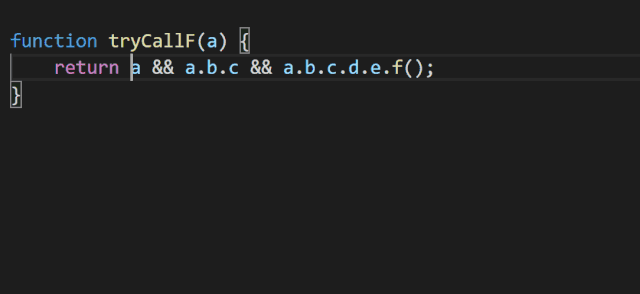https://github.com/microsoft/TypeScript/pull/39135

/** @deprecated */ 支持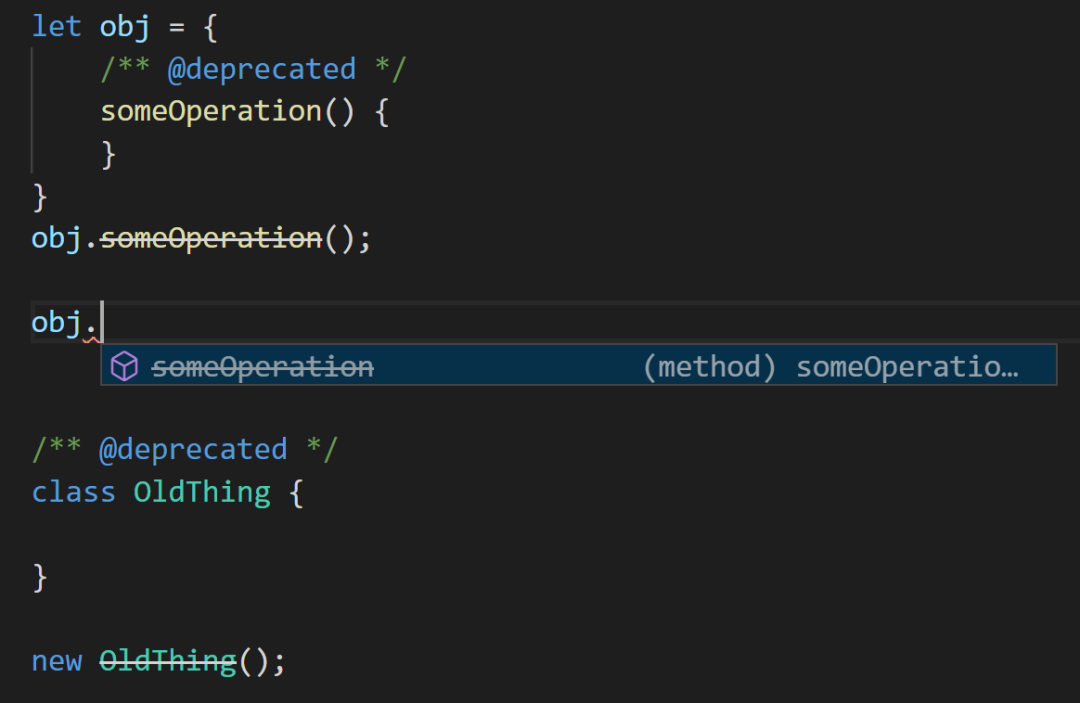https://github.com/microsoft/TypeScript/pull/38523

https://github.com/microsoft/TypeScript/issues/39035

https://github.com/microsoft/TypeScript/issues/37713

TypeScript 4.0 现在可以包含你在 package.json 的 dependencies（和 peerDependencies）字段中列出的包。这些包中的信息仅用于改进自动导入，不会更改类型检查等其他内容。这样就避免了遍历 node_modules 目录的成本，使我们可以为所有带类型的依赖项提供自动导入。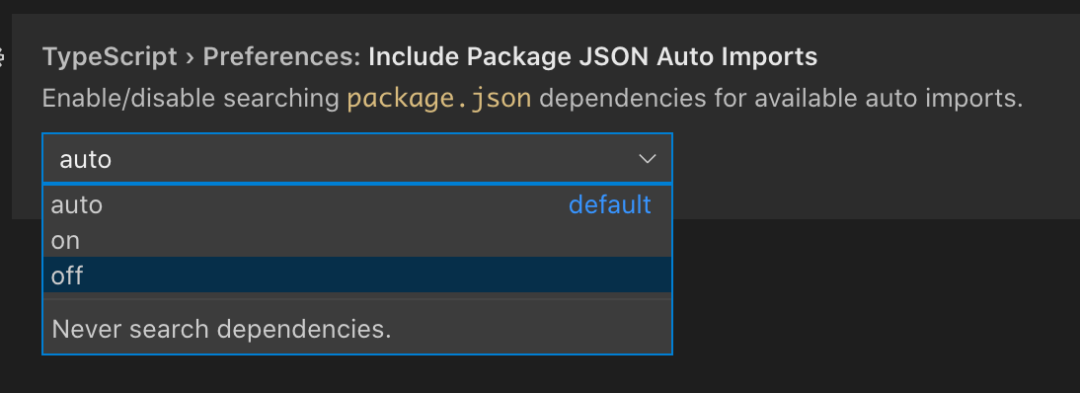https://github.com/microsoft/TypeScript/issues/37812

TypeScript 网站最近被彻底重写了！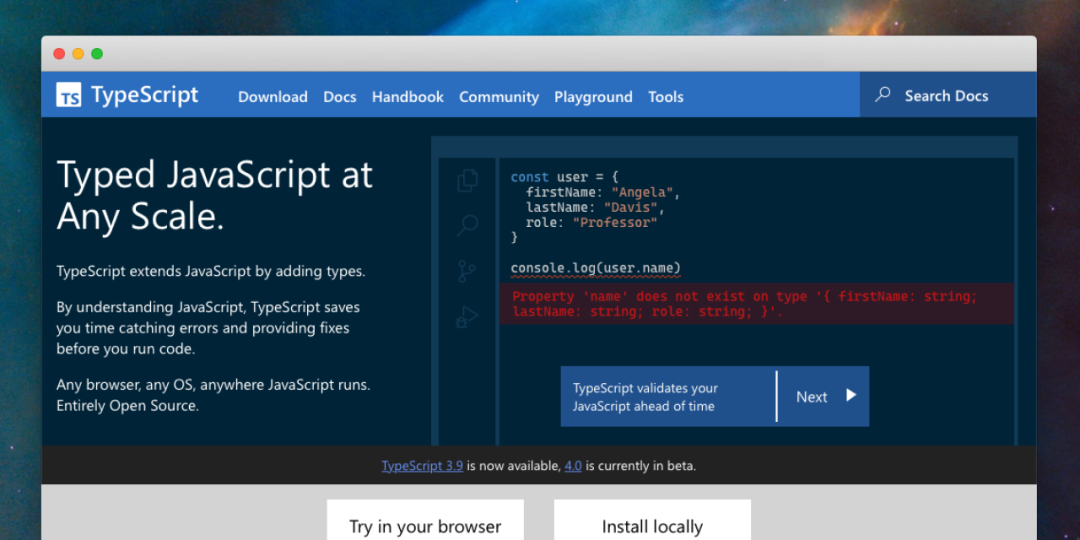《TypeScript 新版网站上线：带来了新的导航机制》

lib.d.ts

``````class Base {    get foo() {        return 100;    }    set foo() {        // ...    }}class Derived extends Base {    foo = 10;//  ~~~// error!// 'foo' is defined as an accessor in class 'Base',// but is overridden here in 'Derived' as an instance property.}

class Base {    prop = 10;}class Derived extends Base {    get prop() {    // ~~~~    // error!    // 'prop' is defined as a property in class 'Base', but is overridden here in 'Derived' as an accessor.        return 100;    }}
``````

delete 的操作数必须是可选的

``````interface Thing {    prop: string;}function f(x: Thing) {    delete x.prop;    // ~~~~~~    // error! The operand of a 'delete' operator must be optional.}
``````

TypeScript 的 Node 工厂用法已弃用

https://github.com/microsoft/TypeScript/pull/35282

TypeScript 4.1 的迭代计划已经上线了，你可以大致了解一下。

https://github.com/microsoft/TypeScript/issues/40124

https://devblogs.microsoft.com/typescript/announcing-typescript-4-0/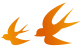End

>> 每满100减50  <<blmius
2年前
MySQL:[Err] 1292 - Incorrect datetime value: ‘0000-00-00 00:00:00‘ for column ‘CREATE_TIME‘ at row 1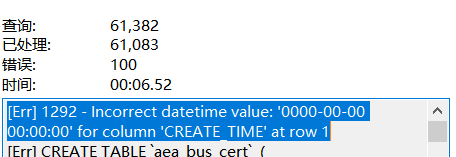Jacquelyn38
2年前
2020年前端实用代码段，为你的工作保驾护航Easter79
2年前
swap空间的增减方法
（1）增大swap空间去激活swap交换区：swapoff v /dev/vg00/lvswap扩展交换lv：lvextend L 10G /dev/vg00/lvswap重新生成swap交换区：mkswap /dev/vg00/lvswap激活新生成的交换区：swapon v /dev/vg00/lvswaphelloworld_34035044
1年前

​在我们用皕杰报表工具设计填报报表时，如何在新增行里自动增加id呢？能新增整数排序id吗？目前可以在新增行里自动增加id，但只能用uuid函数增加UUID编码，不能新增整数排序id。uuid函数说明：获取一个UUID，可以在填报表中用来创建数据ID语法：uuid()或uuid(sep)参数说明：sep布尔值，生成的uuid中是否包含分隔符''，缺省为Wesley13
2年前
Java获得今日零时零分零秒的时间（Date型）
publicDatezeroTime()throwsParseException{    DatetimenewDate();    SimpleDateFormatsimpnewSimpleDateFormat("yyyyMMdd00:00:00");    SimpleDateFormatsimp2newSWesley13
2年前
mysql设置时区
mysql设置时区mysql\_query("SETtime\_zone'8:00'")ordie('时区设置失败，请联系管理员！');中国在东8区所以加8方法二：selectcount(user\_id)asdevice,CONVERT\_TZ(FROM\_UNIXTIME(reg\_time),'08:00','0Wesley13
2年前
00：Java简单了解Stella981
2年前Wesley13
2年前
MySQL部分从库上面因为大量的临时表tmp_table造成慢查询京东云开发者
1年前Easter79
Lv1

2.8k

5

1.2k